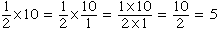My name is Constance and I am thirteen years old (I am a student). The question that I am queering about I don't understand why you do ONE HALF x the base x the width WHEN YOU WANT TO FIND the area of a triangle? My second question is if you multiply one half and 10 together why does it come out as 5? Hi Constance, We were asked thae same question a while ago and you can find an answer in our database. Your second question has to do with the way we write fractions. If you take ten pennies and separate them into two equal sized piles then you have 5 in each pile. That is 10 divided by 2 is 5. We write this as  10/2 = 5. ButI hope this helps Penny Go to Math Central